# 分治（详解残缺棋盘 —— Java 代码实现）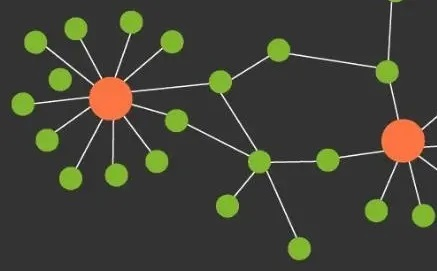# 分治

## 总体思想

• 将要求解的较大规模的问题分割成 k 个更小规模子问题

• 对这 k 个子问题分别求解。如果子问题的规模仍然不够小，则再划分为 k 为子问题，如此递归进行下去，直到问题规模足够小，很容易求出其解为止

• 将求出的小规模的问题的解合并为一个更大规模的问题的解，自底向上逐步求出原来的问题的解

## 使用条件

• 该问题的规模缩小到一定的程度就可以容易地解决

• 该问题可以分解为若干个规模较小的相同问题，即该问题具有最优子结构性质

• 利用该问题分解出的子问题的解可以合并为该问题的解

• 该问题所分解出的各个子问题是相互独立的，即子问题之间不包含公共的子问题

## 基本步骤

divide-and-conquer(P) {  if (|P| <= n0) abhoc(P);  // 解决小规模的问题  divide P into smaller subinstances P1, P2 ,... ,Pk  // 分解问题  for (i = 1; i <= k; i++) {    yi = divide-and-conquer(Pi);  // 递归的解各子问题    return merge(y1, ... ,yk)  // 将各子问题的解合并为原问题的解  }}

## 案例

### 覆盖残缺棋盘

• 在一个 2k×2k 个方格组成的棋盘中，恰有一个方格与其他方格不同，称该方格为一特殊方格，且称该棋盘为一特殊棋盘。

• 用 (n<sup>2</sup>) / 3 个三重格放置在 n × n 的缺陷棋盘上，正好能够覆盖所有方格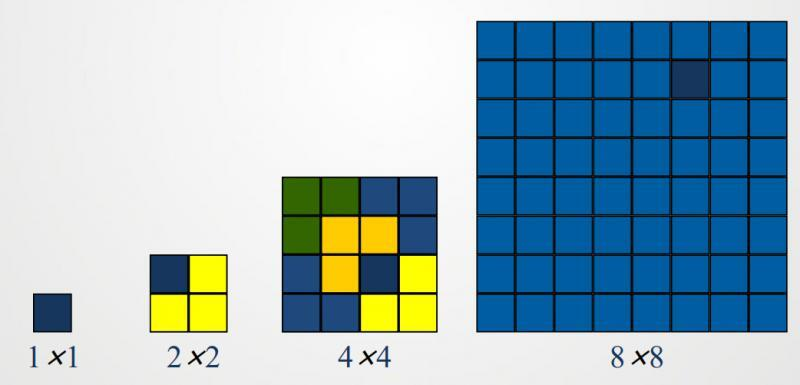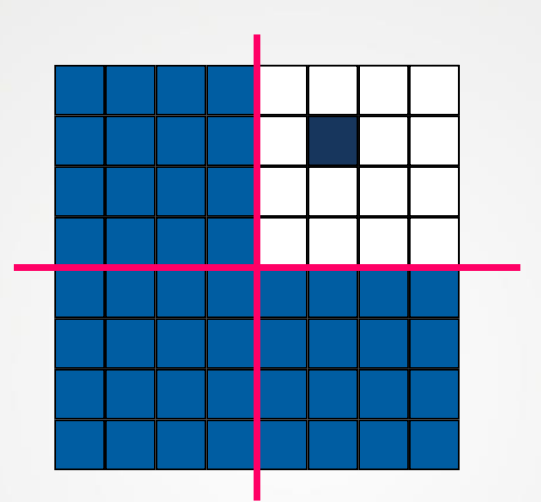• 划分为四个小棋盘

• 其中一个是 4 × 4 缺陷棋盘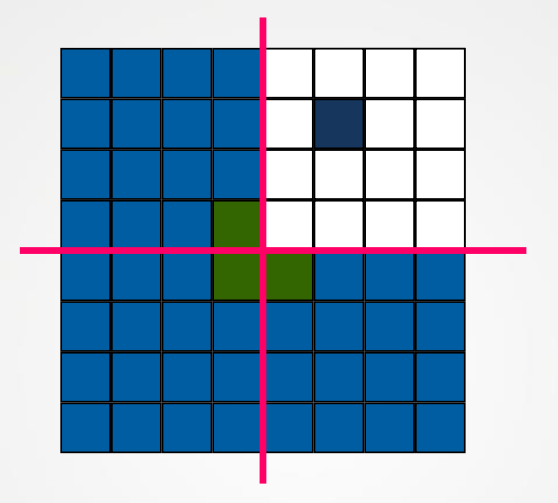• 在其他三个 4 × 4 棋盘都都相邻的拐角上放一个三格板，使它们也成为缺陷棋盘

• 递归地覆盖四个 4×4 缺陷棋盘

• 在其它三个 4 × 4 棋盘都相邻的拐角上放一个三格板，使它们也成为缺陷棋盘。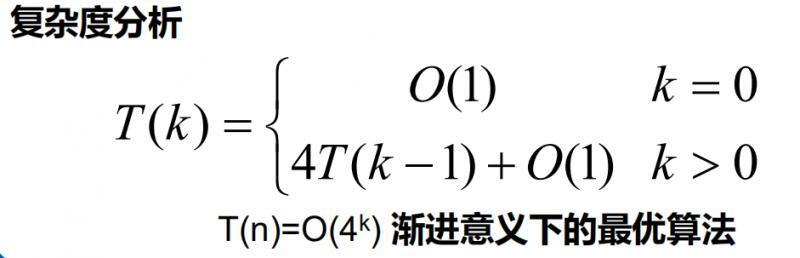Java 代码实现

package Chess;public class Chess {  // 表示棋盘  private int[][] board;  // 表示棋盘的大小为2的多少次方  private int boardSize;  // 棋盘中特殊方格的位置（行号，列号）  private int dr, dc;  private int tile = 1;    public Chess() {    board = new int;    dr = 0;    dc = 0;    boardSize = 0;  }    public Chess(int r, int c, int s) {    int n;    n = (int) Math.pow(2, s);    if (n <= r || n <= c)      System.out.println("初始化参数错误！");    else {      board = new int[n][n];      dr = r;      dc = c;      boardSize = s;    }  }    public void Print() {    for (int i = 0; i < Math.pow(2, this.boardSize); i++) {      for (int j = 0; j < Math.pow(2, this.boardSize); j++) {        System.out.printf("%3d|", this.board[i][j]);      }      System.out.println();    }  }    public static void main(String[] args) {    // 2^2*2^2棋盘, 假设特殊方格位置为（3, 3）    Chess c1 = new Chess(3, 3, 2);    c1.chessBoard(0, 0, c1.dr, c1.dc, (int)Math.pow(2, c1.boardSize));    c1.Print();  }      /**   * @param tr：棋盘左上角方格的行号   * @param tc：棋盘左上角方格的列号   * @param dr：特殊方格所在的行号   * @param dc：特殊棋盘所在的列号   * @param size：2^k, 棋盘的规格为 2^k*2^k   **/  public void chessBoard(int tr, int tc, int dr, int dc, int size) {    if (size == 1) return;        // t: L型骨牌号，s分割棋盘    int t = tile++;    int s = size / 2;        // 覆盖左上角棋盘    if (dr < tr + s && dc < tc + s) {      // 特殊方格在此棋盘中      chessBoard(tr, tc, dr, dc, s);    } else {       // 此棋盘中无特殊方格则用t号L型骨牌覆盖右下角      board[tr + s - 1][tc + s - 1] = t;      // 覆盖其余方格      chessBoard(tr, tc, tr + s - 1, tc + s - 1, s);    }        // 覆盖右上角子棋盘    if (dr < tr + s && dc >= tc + s) {      // 特殊方格在此棋盘中      chessBoard(tr, tc + s, dr, dc, s);    } else {      // 无特殊方格，用t号骨牌覆盖左下角      board[tr + s - 1][tc + s] = t;      chessBoard(tr, tc + s, tr + s - 1, tc + s, s);    }        // 覆盖左下角棋盘    if (dr >= tr + s && dc < tc + s) {      chessBoard(tr + s, tc, dr, dc, s);    } else {      // 无特殊方格，用t号骨牌覆盖右上角      board[tr + s][tc + s - 1] = t;      chessBoard(tr + s, tc, tr + s, tc + s - 1, s);    }        // 覆盖右下角棋盘    if (dr >= tr + s  && dc >= tc + s) {      // 特殊方格在此棋盘中      chessBoard(tr + s, tc + s, dr, dc, s);    } else {      // 无特殊方格，用t号骨牌覆盖左上角      board[tr + s][tc + s] = t;      chessBoard(tr + s, tc + s, tr + s, tc + s, s);    }  }}

  2|  2|  3|  3|  2|  1|  1|  3|  4|  1|  5|  5|  4|  4|  5|  0|

### 大整数的乘法

• 小学的方法：效率太低 O(n2)

• 分治法：

•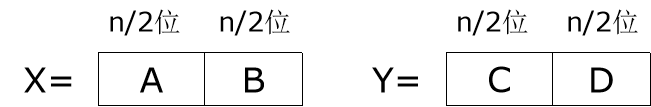• X = A × 2<sup>n/2</sup> + BY = C × 2<sup>n/2</sup> + DXY = (A × 2<sup>n/2</sup> + B)(C × 2<sup>n/2</sup> + D)     =AC × 2<sup>n</sup> + (AD + CB) × 2<sup>n/2</sup> + BD

•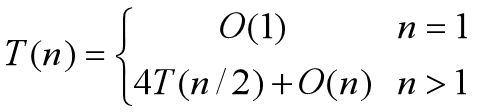• 实质上没有改进再次进行改进：XY = AC × 2<sup>n</sup> + (AD + CB) × 2<sup>n/2</sup> + BD     =AC × 2<sup>n</sup> + ((A - B)(D - C) + AC + BD) × 2<sup>n/2</sup> + BD 这样只需进行 3 次 n/2 位乘法

•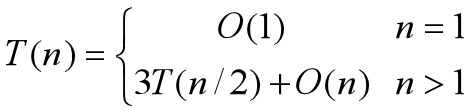• T(n) = O(n<sup>log3</sup>) = O(n<sup>1.59</sup>) （有了较大的改进

### Strassen 矩阵乘法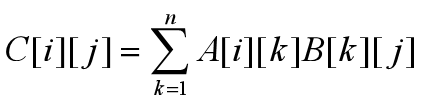• 将矩阵 A、B 和 C 中每一矩阵都分成 4 个大小相等的子矩阵，则 C = AB 可写为：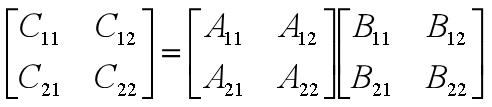由此可得：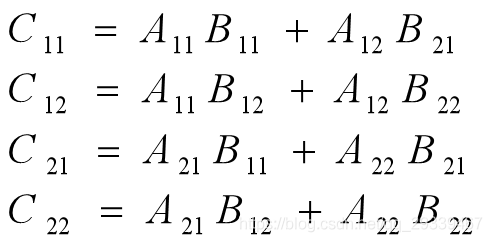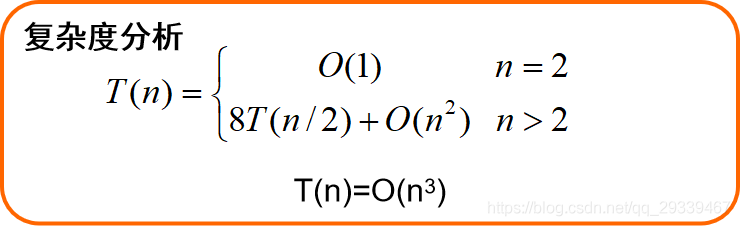实际复杂度还是没有变，仍然为 O(n<sup>3</sup>)

• 为了降低时间复杂度，要减少乘法的次数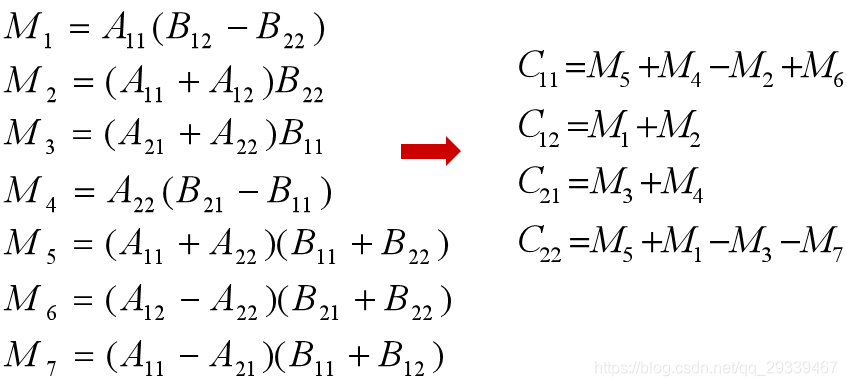这样，复杂度得到了改进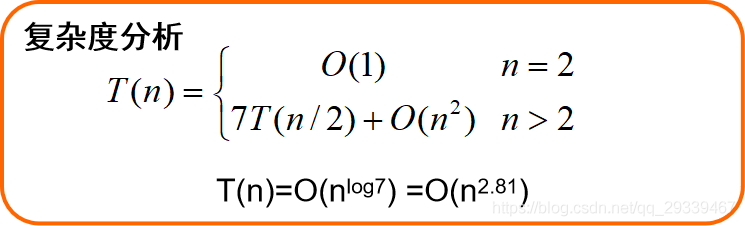## 评论